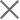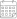Subscribe

### Indexes

April 2018 | Electricity Market Review30 April 2018

In April 2018, Georgian power plants generated 1,064 mln. KWh of electricity. This represents a 30% increase in total generation, compared to the previous year (in 2017, total generation in April was 817.2 mln. kWh). The increase in generation on a yearly basis mainly comes from an increase in hydro power generation (more details below).

On a monthly basis, generation increased by 7% (in March 2018, total generation was 997 mln. kWh).

The share of electricity produced by renewable sources increased to 97% of total generation (1028 mln kWh), while thermal power generation decreased in comparison to March 2018, accounting for 3% of total generation (35 mln. kWh).

Consumption of electricity on the local market was 981 mln. kWh (+5% compared to April 2017, and -12% with respect to March 2018). In April 2018, generation exceeded total consumption by 21 mln - 2% of the total amount generated (compared to 119 mln kWh and a 12% deficit in total generation for March 2018).

Among the different sources of electricity, hydropower became even more dominant. Specifically, in April 2018, hydropower (HPP) generation amounted to 1,019 mln. kWh (96% of total), wind power (WPP) was 9 mln. kWh (1% of total), and thermal power (TPP) was 35 mln. kWh (3% of total) (Figure 2). Among hydropower generators, large (regulatory) HPPs produced 52% (532 mln. kWh) of electricity, while seasonal and small HPPs produced 40% (407 mln. kWh) and 8% (80 mln. kWh), respectively (see the report).